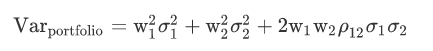# Correlation Coefficient and Variance

Which one of the following statements about correlation is NOT correct?

A)

Potential benefits from diversification arise when correlation is less than +1.

B)

If the correlation coefficient were -1, a zero variance portfolio could be constructed.

C)

If the correlation coefficient were 0, a zero variance portfolio could be constructed.

I had to think this one through, is this logic correct? Since the formula for Variance isand we want 0 variance, that would mean we need the third term 2w1*w2*Corr1,2*stdev1*stdev2 to equal negative some number. That way we can add the other two positive terms to maybe come up with zero variance?

The correct answer is B, I forgot to mention above.